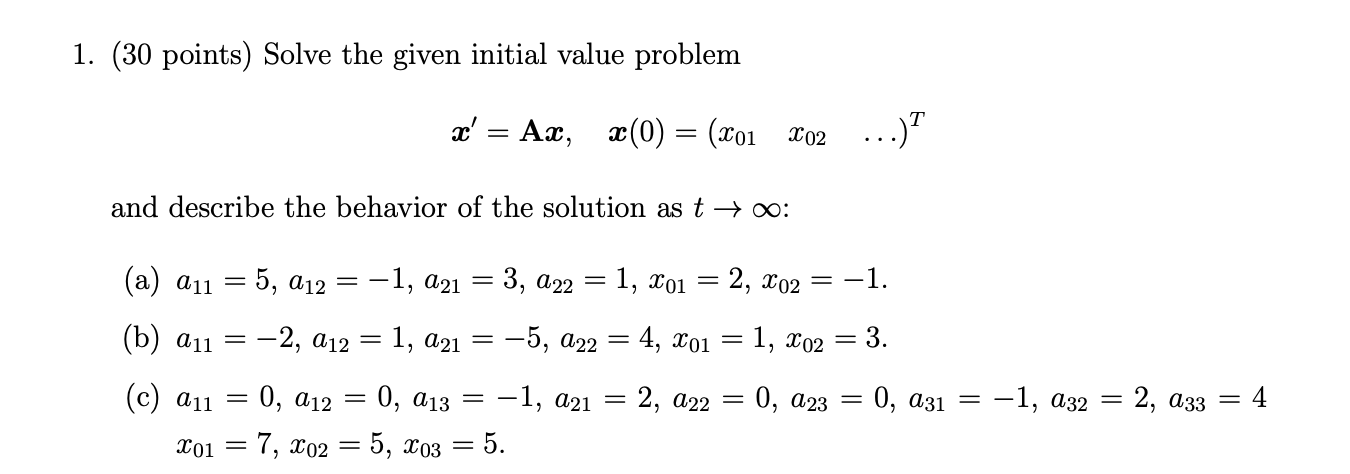# (Solved): Please answer question b or c. Ignore question a.  1. (30 points) Solve the given initial ...Please answer question b or c. Ignore question a.

1. (30 points) Solve the given initial value problem $\boldsymbol{x}^{\prime}=\mathbf{A} \boldsymbol{x}, \quad \boldsymbol{x}(0)=\left(\begin{array}{lll} x_{01} & x_{02} & \ldots \end{array}\right)^{T}$ and describe the behavior of the solution as $$t \rightarrow \infty$$ : (a) $$a_{11}=5, a_{12}=-1, a_{21}=3, a_{22}=1, x_{01}=2, x_{02}=-1$$. (b) $$a_{11}=-2, a_{12}=1, a_{21}=-5, a_{22}=4, x_{01}=1, x_{02}=3$$. (c) $$a_{11}=0, a_{12}=0, a_{13}=-1, a_{21}=2, a_{22}=0, a_{23}=0, a_{31}=-1, a_{32}=2, a_{33}=4$$ $$x_{01}=7, x_{02}=5, x_{03}=5$$

We have an Answer from Expert